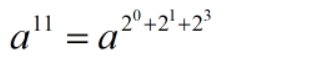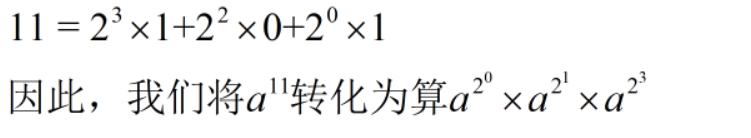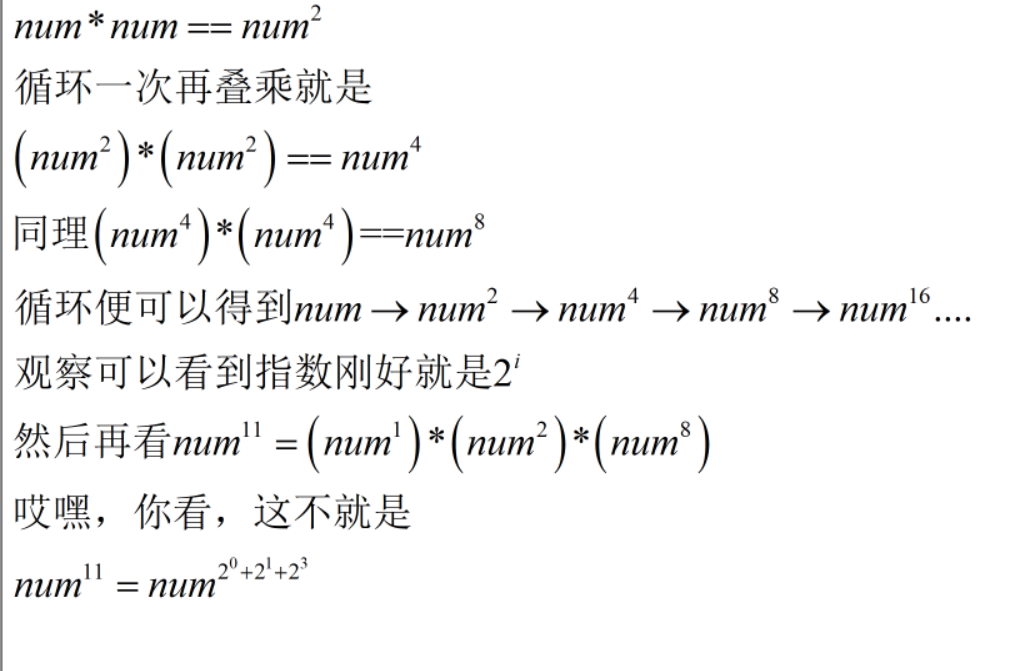• 取模运算与取余运算的相同点 公式相同： 取模运算： A mod B = A - (A / B) * B 取余运算： A rem B = A - (A / B) * B 取模运算与取余运算的不同点 对于 A / B 的定义不同： 取模运算在计算 A / B 的值时，向...
取模运算与取余运算的相同点
公式相同： 取模运算： A mod B = A - (A / B) * B 取余运算： A rem B = A - (A / B) * B
取模运算与取余运算的不同点
对于 A / B 的定义不同： 取模运算在计算 A / B 的值时，向负无穷方向取整(floor()函数) 取余运算在计算 A / B 的值时，向0 方向取整(fix()函数)  举例说明： -3 / 2 = -1.5 取模运算时，将 -1.5 向负无穷方向取整，得到 -2 取余运算时，将 -1.5 向0方向取整，得到 -1 所以， -3 mod 2 = -3 - (-2) * 2 = 1 -3 rem 2 = -3 - (-1) * 2 = -1
总结
取余运算与取模运算在两数为同号时，结果相同；当两数异号时，结果不同。
展开全文java
• 在一些竞赛中，可能会遇到指数型的数据取模问题，这个时候直接用int或者long long 储存，就非常有可能超出计算机整数的存取范围(哈哈，一般都会超，毕竟是竞赛！！！)，从而数据出错，所以我们就引出今...
最近在牛客第一次碰到有关快速幂的知识，在此记录加深理解一下。大佬勿进，我怕《《 》》
快速取幂的用途
在一些竞赛中，可能会遇到指数型的数据取模问题，这个时候直接用int或者long long 储存，就非常有可能超出计算机整数的存取范围(哈哈，一般都会超，毕竟是竞赛！！！)，从而数据出错，所以我们就引出今天的主角色 快速幂取模。这种方法在时间和空间都做了尽可能的优化，非常好用哦！
快速幂取模的思路分析
基本理论是离散数学的知识*(数学很重要!）* 有一个引理我们需要清楚的：积的取余等于取余的积的取余。 公式：(ab)%c = (a%c)(b%c)%c 核心思想是将大数幂运算拆解成相应的乘法运算，利用上述式子，始终将我们的运算的数据量控制在c的范围下。
以求a的b次方来介绍 把b转换成二进制数。 例如11的二进制是1011对二进制的位运算，在此就不赘述，有问题的同学请自行百度，这里我们需要用到“&”与“>>”运算符。
先来一段具体代码，由代码来讲解
ll binaryPow(ll num, ll k)
{
ll ans = 1;
while(k>0)
{
if(k&1)
ans = ans*num%mod;//如果k的二进制位不是0，那么就会进行该步
num = num*num%mod;//不断加倍
k >>=1; //相当于每次除以2，用二进制看，不断的遍历k的二进制位
}
return ans;
}
在上面的代码中，k&1意思就是取k的二进制的最末位，11的二进制数为1011，第一次循环，取的是最右边的1，以此类推。k>>=1意思就是k右移一位，删去最低位置。 接下来我来说说while循环中的原理。 以num^11为例子，k = 11 k 的二进制 1011。 我们要理解num = num * num；这一步的作用。哎嗨，快速幂就讲解完了。希望大家可以共同进步。另外注明一点，代码%mod也是为了防止溢出，这是从下面一道训练题想出来的。 下面贴出来一道例题。 题目链接 https://ac.nowcoder.com/acm/contest/3003/G
#include <cstdio>
#include <iostream>
#include <algorithm>
#include <cstring>
#include <cmath>
using namespace std;
typedef long long ll;
const int maxn = 200005;
const int mod = 1e9+10;
typedef long long ll;
ll binaryPow(ll num, ll k)
{
ll ans = 1;
while(k>0)
{
if(k&1)
ans = ans*num%mod;
num = num*num%mod;
k >>=1;
}
return ans;
}

int main()
{
ios::sync_with_stdio(false);
int t;
cin >> t;
while (t--)
{
long long a, b, c, d, e, f, g;
cin >> a >> b >> c >> d >> e >> f >> g;
int sum = 0;
int m = 1e9 + 7;
if (binaryPow(a,d)+binaryPow(b,e)+binaryPow(c,f) == g)
{
cout << "Yes" << endl;
}
else
{
cout << "No" << endl;
}
}
return 0;
}
就这样吧，溜走。
展开全文• 整数部分，把十进制转成二进制一直分解至商数为0。读余数从下读到上，即是二进制的整数部分数字。 小数部分，则用其乘2，取其整数部分的结果，再用计算后的小数部分依此重复计算，算到小数部分全为0为止，之后读所有...
十进数转成二进数
整数部分采用 "除2取余，逆序排列"法。
整数部分，把十进制转成二进制一直分解至商数为0。读余数从下读到上，即是二进制的整数部分数字。 小数部分，则用其乘2，取其整数部分的结果，再用计算后的小数部分依此重复计算，算到小数部分全为0为止，之后读所有计算后整数部分的数字，从上读到下。 将59 转成二进制：
整数部分：
59 ÷ 2 = 29 ... 1
29 ÷ 2 = 14 ... 1
14 ÷ 2 =  7 ... 0
7 ÷ 2 =  3 ... 1
3 ÷ 2 =  1 ... 1
1 ÷ 2 =  0 ... 1

十进制的59转化二进制为111011
二进位转成十进位
方法：“按权展开求和”，该方法的具体步骤是先将二进制的数写成加权系数展开式，而后根据十进制的加法规则进行求和  。
【例】：规律：个位上的数字的次数是0，十位上的数字的次数是1，…，依次递增，而十分位的数字的次数是-1，百分位上数字的次数是-2，…，依次递减。
二进制的1011对应十进制的11
使用位运算(&)代替取模运算(%)
符号描述运算规则&与两个位都为1时，结果才为1|或两个位都为0时，结果才为0^异或两个位相同为0，相异为1~取反0变1，1变0<<左移各二进位全部左移若干位，高位丢弃，低位补0>>右移各二进位全部右移若干位，对无符号数，高位补0，有符号数，各编译器处理方法不一样，有的补符号位（算术右移），有的补0（逻辑右移）
位运算(&)效率要比取模运算(%)高很多，主要原因是位运算直接对内存数据进行操作，不需要转成十进制，因此处理速度非常快。
a % b == a & (b - 1)

前提：b 为 2^n
来源自 HashMap 中的 indexFor 方法：
static int indexFor(int h, int length) {
return h & (length-1);//h%length
}

这个方法是使用哈希值对链表数组的长度取模，得到值所在的索引位置，里面使用位运算（&）代替普通的（%）运算。
展开全文• 运算应用口诀  清零取反要用与，某位置一可用或 若要取反和交换，轻 轻松松用异或 移位运算 要点 1 它们都是双目运算符，两个运算分量都是整形，结果也是整形。  2 "  3 ">>"右移：右边的位被挤掉。...


位运算应用口诀
清零取反要用与，某位置一可用或

若要取反和交换，轻 轻松松用异或

移位运算

要点 1 它们都是双目运算符，两个运算分量都是整形，结果也是整形。

2 "<<" 左移：右边空出的位上补0，左边的位将从字头挤掉，其值相当于乘2。

3 ">>"右移：右边的位被挤掉。对于左边移出的空位，如果是正数则空位补0，若为负数，可能补0或补1，这取决于所用的计算机系统。

4 ">>>"运算符，右边的位被挤掉，对于左边移出的空位一概补上0。

(1) 按位与-- &

(2) 按位或-- |

(3) 位异或-- ^

2 不引入第三变量，交换两个变量的值 (设 a=a1,b=b1)

目标           操作              操作后状态

a=a1^b1         a=a^b              a=a1^b1,b=b1

b=a1^b1^b1      b=a^b              a=a1^b1,b=a1

a=b1^a1^a1      a=a^b              a=b1,b=a1

二进制补码运算公式：

-x = ~x + 1 = ~(x-1)

~x = -x-1

-(~x) = x+1

~(-x) = x-1

x+y = x - ~y - 1 = (x|y)+(x&y)

x-y = x + ~y + 1 = (x|~y)-(~x&y)

x^y = (x|y)-(x&y)

x|y = (x&~y)+y

x&y = (~x|y)-~x

x==y:    ~(x-y|y-x)

x!=y:    x-y|y-x

x< y:    (x-y)^((x^y)&((x-y)^x))

x<=y:    (x|~y)&((x^y)|~(y-x))

x< y:    (~x&y)|((~x|y)&(x-y))//无符号x,y比较

x<=y:    (~x|y)&((x^y)|~(y-x))//无符号x,y比较

应用举例

(1) 判断int型变量a是奇数还是偶数

a&1   = 0 偶数

a&1 =   1 奇数

(2) 取int型变量a的第k位 (k=0,1,2……sizeof(int))，即a>>k&1

(3) 将int型变量a的第k位清0，即a=a&~(1<<k)

(4) 将int型变量a的第k位置1， 即a=a|(1<<k)

(5) int型变量循环左移k次，即a=a<<k|a>>16-k   (设sizeof(int)=16)

(6) int型变量a循环右移k次，即a=a>>k|a<<16-k   (设sizeof(int)=16)

(7) 整数的平均值

对于两个整数x,y，如果用 (x+y)/2 求平均值，会产生溢出，因为 x+y 可能会大于INT_MAX，但是我们知道它们的平均值是肯定不会溢出的，我们用如下算法：

int average(int x, int y)   //返回X,Y 的平均值

{

return (x&y)+((x^y)>>1);

}

(8)判断一个整数是不是2的幂,对于一个数 x >= 0，判断他是不是2的幂

boolean power2(int x)

{

return ((x&(x-1))==0)&&(x!=0)；

}

(9)不用 temp交换两个整数

void swap(int x , int y)

{

x ^= y;

y ^= x;

x ^= y;

}

(10) 计算绝对值

int abs( int x )

{

int y ;

y = x >> 31 ;

return (x^y)-y ;        //or: (x+y)^y

}

(11) 取模运算转化成位运算 (在不产生溢出的情况下)

a % (2^n) 等价于 a & (2^n - 1)

(12)乘法运算转化成位运算 (在不产生溢出的情况下)

a * (2^n) 等价于 a<< n

(13)除法运算转化成位运算 (在不产生溢出的情况下)

a / (2^n) 等价于 a>> n

例: 12/8 == 12>>3

(14) a % 2 等价于 a & 1

(15) if (x == a) x= b;

else x= a;

等价于 x= a ^ b ^ x;

(16) x 的 相反数 表示为 (~x+1)


展开全文• 好的标题就告诉我们该来的还是会来，学了十几年十进制现在告诉我要学二进制，但是这个东西很重要很重要，所以还是要重点记 part 1：什么是二进制二进制，是计算技术中广泛采用的一种数制，由德国数理哲学大师...c++
• //求字符串中的二进制和 //比如 a="11" //b= "1" //sum= 100 // 目前有两种方式来做，第一种就是用模拟二进制加法的方式，第二种就是将二进制转换成十进制 // 然后相加之后，转回二进制的方法 第一种: 二进制加法...leetcode 算法 golang
• 首先来看下取余与取模的区别： ...求模运算和求余运算在第一步不同: 取余运算在取c的值时，向0 方向舍入(fix()函数)；而取模运算在计算c的值时，向负无穷方向舍入(floor()函数)。 例如：计算-7 Mod 4 那么：a = -7；
• 运算总结取模取余tags:运算总结via:http://blog.csdn.net/black_ox/article/details/46411997Summary: 位运算应用口诀 清零取反要用与，某位置一可用或 若要取反和交换，轻 轻松松用异或 移位运算 要点 1 它们都是...
• 运算应用口诀清零取反要用与，某位置一可用或 若要取反和交换，轻 轻松松用异或 移位运算 要点 1 它们都是双目运算符，两个运算分量都是整形，结果也是整形。 2 "<<" 左移：右边空出的位上补0，左边的...
• 本题大意：求a1的b1次方加a2的b2次方一直加到an的bn次方，用他们幂的和对一个数x取余，把结果输出！ 参考出处：http://www.cnblogs.com/yan-boy/archive/2012/11/29/2795294.html（感谢原作者！） 矩阵的快速幂...acm 算法
• 二进制运算一，前言二，取整运算三，七种位运算1，&：与运算，遇0则02，|： 或运算，遇1则1。3，~：取反，0变1，1变04，^：异或运算5， <<<<<< ：算数左移，低位填充0，高位舍弃6， >>...补码
• ## 逆向-取模运算

千次阅读 2020-04-15 14:15:29
除法终于整理结束了，这篇开始整理取模运算，对于取模运算来说，个别的情况还是需要使用除法的，所以除法运算的基础还是得牢靠。 取模也叫取余，表达为 a % b。下面需要对b的情况进行区分来分析 1. 变量 2. 常量 ...
• 优化方法：将取模运算转化为位运算，加快执行。 理论基础： 命题：x % 2^N == x & (2^N - 1) 证明： 因为 (2^N-1)的二进制的低N位全是1，其余位全是0， 所以 (x & (2^N-1))是x的二进制的低N位的值， ...
• * 使用位运算实现 加减乘除 取模 * 原理：加法原则：对应位置进行加和，若果有进位，则加到到高位中。 * 那么使用位运算代替加法，要解决两个问题： * 1、如何计算进位 * 二进制中出现进位的形式只有1+1，可以...java
• > 有些人2^n-1 正好二进制位全是1，类似这种1111111，这样与运算hash冲突才能降到到最低，但是直接取模不是一样的能均匀分布么？ 这里关键的问题就在于究竟是不是位运算取模运算快很多了： ...java 操作系统
• 之前看HashMap源码时，总说HashMap数组大小要用2的n次幂，取模时用到的位运算，这样HashMap取模才会很快，也就知道了这个特性，没有去专门了解过，为什么用2的n次幂，可以用位运算取模；由于最近看一些框架底层...java
• 二进制，位运算，移位运算 1.二进制 对于原码, 反码, 补码而言, 需要注意以下几点: (1).Java中没有无符号数, 换言之, Java中的数都是有符号的; (2).二进制的最高位是符号位, 0表示正数, 1表示负数; (3).正数的...结构 java
• 在计算散列位置时 k = j - 1 & i ，理论上是将hash值对散列表长度 j （默认长度 16）取模，实际则转换成了与运算。 抽象成计算式：X % 2n = X & (2n - 1)性能优化
• ## 二进制位运算

千次阅读 2016-01-30 13:48:59
位权：数制中每一固定位置对应的单位值，也就是某一位上的“1”所表示的数值的大小，称为该位的位权. 比如10进制数：1234， 个位位权1*10^0=1; 十位位权1*10^1=10;... 二进制和十进制的转换：比如67这个数java
• 快速幂运算 ...二进制拆分 快速幂-反复平方法 代码如下： long long qpow (long long a,long long b){ long long result=1; while (b){ if (b&1) result*=a; b>>=1; a=a*a; } return resul算法
• if(b & 1)//&是位运算，b&1表示b在二进制下最后一位是不是1，如果是： ans *= base;//把ans乘上对应的a^(2^n) base *= base;//base自乘，由a^(2^n)变成a^(2^(n+1)) b >>= 1;//位运算，b右移一位，如101变成10...
• 将一个长度最多为30位数字的十进制非负整数转换为二进制数输出。 输入 多组数据，每行为一个长度不超过30位的十进制非负整数。 （注意是10进制数字的个数可能有30个，而非30bits的整数） 输出 每行输出对应的...
• 【题目描述】 输入三个正整数 a,b,c 计算 a^b mod c。...将指数分解为二进制，再遍历每一位，每次底数a=a*a，当本位为1时ans*=a。取模的地方在更新ans之前ans和a都要取模，底数相乘之前a也要取模
• 输出一行一个字符串 a^b mod p=s，其中 a,b,p 分别为题目给定的值， s 为运算结果。 输入输出样例 输入 #1复制 2 10 9 输出 #1复制 2^10 mod 9=7 说明/提示 样例解释 2^10=1024,1024mod9=7。 数据规模与约定对于100%...python 算法
• ps：在c/c++,c#,java,php中%是取余运算，python中是取模运算。 此外，余数在数学中的定义是始终大于等于0的。 百度百科上面说数学的余数是用取模公式，那暂且相信吧。 · （左移） 2 , 2的二进制为10，左移两位...python
• 1. 为什么会有原码反码补码？ 2. 二进制运算 3. 二进制运算与HashMapjava hashmap jdk 补码...